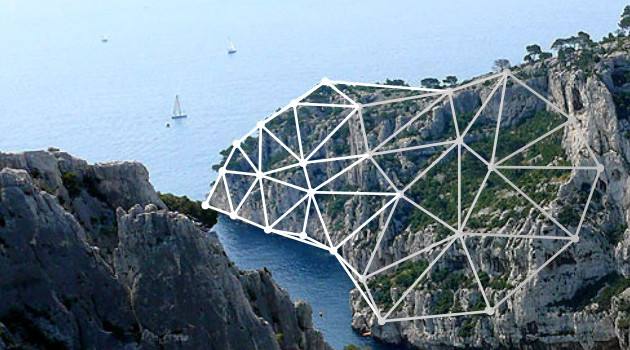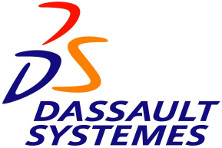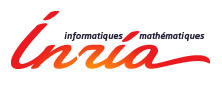# JGA 2013

December 16 - 20, CIRM (Luminy)

The conference is aimed at researchers and students interested in the applicative and algorithmic aspects of geometry and topology. The format features mini courses as well as contributed talks and time for discussion. For this year, the course topics include topological aspects of the motion planning problem, tropical geometry, image processing and approximation algorithms for clustering.The schedule and the program are now available.

##Mini-courses##

##Invited talk##

• Benjamin Burton (The University of Queensland, Australia)
Exploring parameterised complexity in computational topology

##Contributed talks##

• Thomas Bonis, INRIA Geometrica,
Topological pooling slides

• Mickaël Buchet, INRIA Geometrica,
Analyse de champs scalaires avec bruit aberrant slides

• Raphaelle Chaine, LIRIS, Université Lyon 1,
Practical reduction of edge flip sequences in two-dimensional triangulations

• David Cohen-Steiner, INRIA Geometrica,
Improved bounds on higher eigenvalues of graphs

• Louis Cuel, Université de Grenoble - Université de Chambéry
Normals, curvature and sharp feature estimation using order-k Voronoi covariance slides

• Julie Digne, CNRS - Université Lyon 1,
Self-similarity for accurate compression of point sampled surface slides

• Ross Hemsley, INRIA Geometrica
Cone Walk: Navigating a random Delaunay triangulation slides

• Anatolii Kostrygin, 'Ecole polytechnique
Periodic planar straight-frame graph drawings with polynomial resolution slides

• Manish Mandad, INRIA Titane
TBA

• Clément Maria, INRIA Geometrica
The compressed annotation matrix: an efficient data structure for computing persistent cohomology slides

• Clément Maria, INRIA Geometrica
Multi-field persistent homology slides

• Quentin Mérigot, CNRS - Université de Grenoble,
On the reconstruction of convex sets from random normal measurements slides

• Arnaud de Mesmay, École Normale Supérieure Paris,
Discrete systolic inequalities and decompositions of triangulated surfaces slides

• Bertrand Michel, UPMC
Convergence rates for persistence diagram estimation in Topological Data Analysis slides

• Viorica Patraucean, INRIA Geometrica
Mirror-symmetry in images and 3D shapes slides

• Mael Rouxel-Labbé, INRIA Geometrica
Anisotropic Delaunay meshes of surfaces slides

• Régis Straubhaar, INRIA Geometrica
Numerical optimization of Dirichlet-Laplace eigenvalues on domains in surfaces slides

• Boris Thibert, Université de Grenoble
Minkowski-type problems and optimal transport slides

• Rémy Thomasse
A convex body with chaotic hull

## Contact

David Cohen-Steiner

Quentin Mérigot